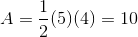Basic Geometry : How to find the area of a right triangle

Example Questions

Example Question #11 : How To Find The Area Of A Right Triangle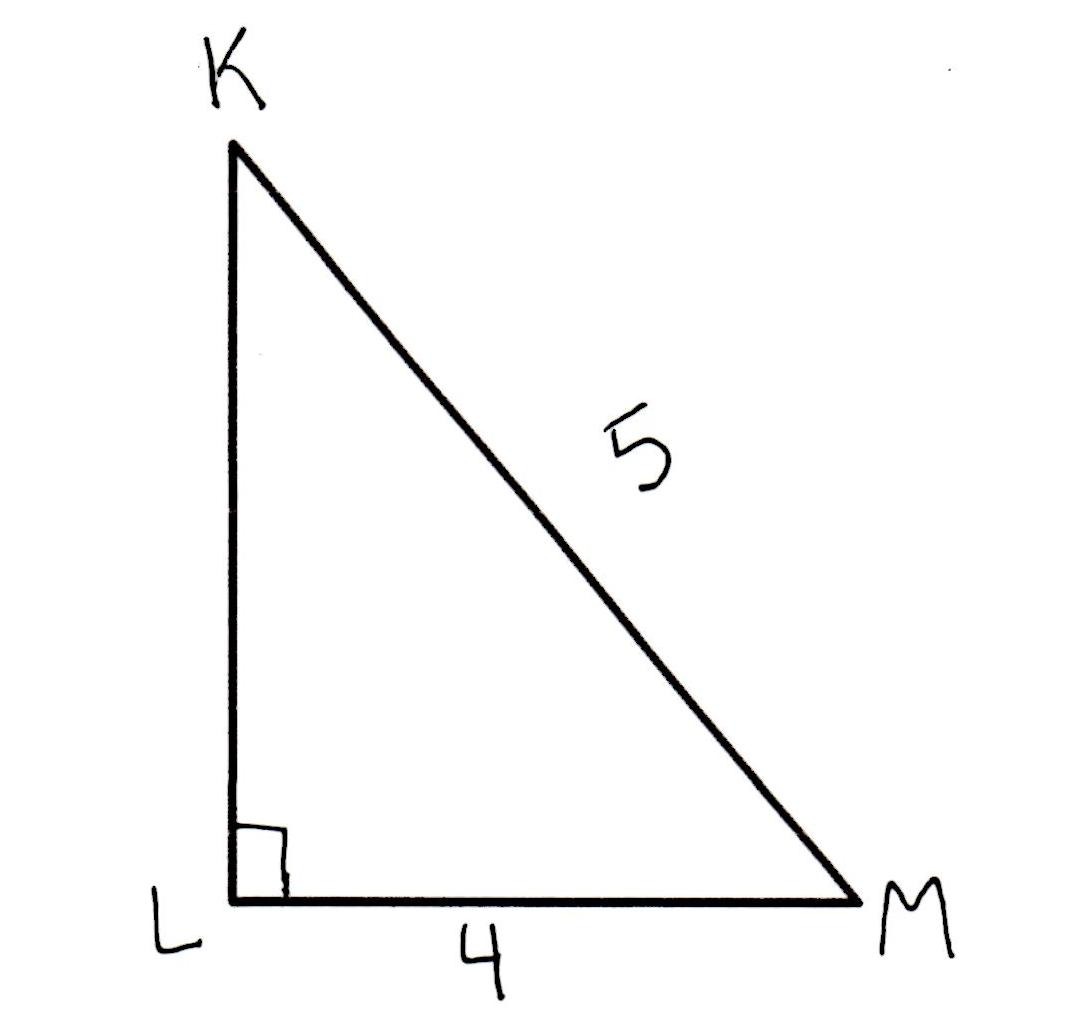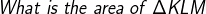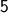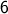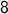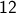Explanation: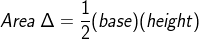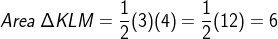Example Question #12 : How To Find The Area Of A Right Triangle

What is the area of a right triangle with a height of 5 inches and a base of 3 inches?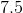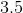Explanation:

To find the area of a triangle, multiply the base by the height, then divide by 2.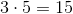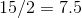Example Question #13 : How To Find The Area Of A Right Triangle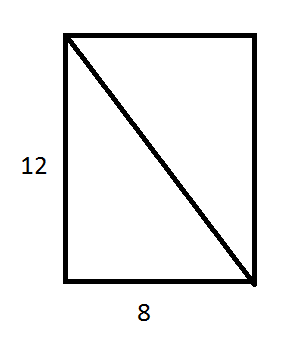Find the area of ONE of the triangles formed by the diagonal.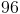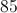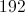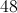Explanation:

To find the area of a right triangle, use the formula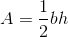, whereis the area of the triangle,is the length of the triangle's base, andis the triangle's height.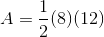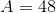Example Question #10 : Right Triangles

A right triangle has a total perimeter of 12, and the length of its hypotenuse is 5. What is the area of this triangle?

12

10

3

15

6

6

Explanation:

The area of a triangle is denoted by the equation 1/2 b x h.

b stands for the length of the base, and h stands for the height.

Here we are told that the perimeter (total length of all three sides) is 12, and the hypotenuse (the side that is neither the height nor the base) is 5 units long.

So, 12-5 = 7 for the total perimeter of the base and height.

7 does not divide cleanly by two, but it does break down into 3 and 4,

and 1/2 (3x4) yields 6.

Another way to solve this would be if you recall your rules for right triangles, one of the very basic ones is the 3,4,5 triangle, which is exactly what we have here

Example Question #6 : How To Find The Area Of A Right Triangle

The ratio for the side lengths of a right triangle is 3:4:5. If the perimeter is 48, what is the area of the triangle?

96

240

108

50

48

96

Explanation:

We can model the side lengths of the triangle as 3x, 4x, and 5x. We know that perimeter is 3x+4x+5x=48, which implies that x=4. This tells us that the legs of the right triangle are 3x=12 and 4x=16, therefore the area is A=1/2 bh=(1/2)(12)(16)=96.

Example Question #67 : Act Math

The length of one leg of an equilateral triangle is 6. What is the area of the triangle?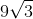Explanation: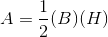The base is equal to 6.

The height of an quilateral triangle is equal to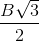, whereis the length of the base.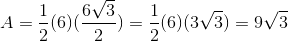Example Question #68 : Act Math

Find the area of the following right triangle to the nearest integer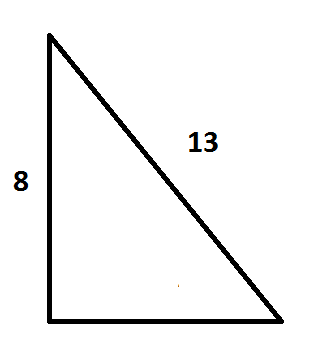Note: The triangle is not necessarily to scale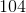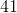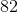Explanation:

The equation used to find the area of a right triangle is: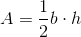where A is the area, b is the base, and h is the height of the triangle. In this question, we are given the height, so we need to figure out the base in order to find the area. Since we know both the height and hypotenuse of the triangle, the quickest way to finding the base is using the pythagorean theorem,. a = the height, b = the base, and c = the hypotenuse.

Using the given information, we can write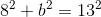. Solving for b, we get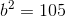or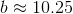. Now that we have both the base and height, we can solve the original equation for the area of the triangle.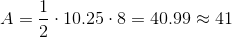Example Question #14 : How To Find The Area Of A Right Triangle

Find the area of this right triangle: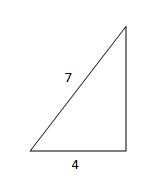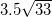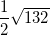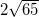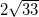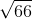Explanation:

The area of a triangle is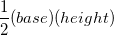. For a right triangle, we can use the two legs as the base and the height regardless of orientation. Right now we only know one of the legs, and we can solve for the other using Pythagorean Theorem: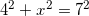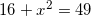subtract 16 from both sides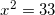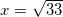we can see that all of the answers are in radical form, so we do not have to simplify this to a crazy decimal.

Now we have our "base" and "height" and we can plug them into the formula to find the area: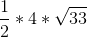half of 4 is 2, so our answer is, which we can't simplify any more.

Example Question #15 : How To Find The Area Of A Right Triangle

Find the area of this right triangle: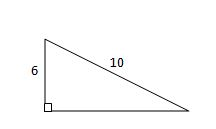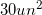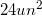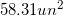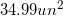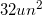Explanation:

The area of a triangle is found using the formula. For a right triangle, we can use the two legs as the base and height regardless of the orientation of the triangle.

In this case, we only know one leg, but we can figure out the other by using the Pythagorean Theorem: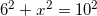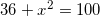subtract 36 from both sides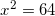take the square root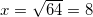Now that we have our "base" and "height," we can substitute them into the formula and find the area: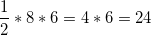Example Question #16 : How To Find The Area Of A Right Triangle

Find the area of a right triangle whose perpendicular sides are of lengthsand.Explanation:

To find area of this triangle, you must use the formula indicated below.whereis base andis height. Thus: